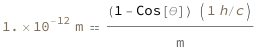# Compton Shift

Compton scattering is the inelastic scattering of a photon by a charged particle, usually an electron. It results in a decrease in energy (increase in wavelength) of the photon, called the Compton effect. Part of the energy of the photon is transferred to the recoiling electron.

The Compton wavelength shift equals 1 minus the cosine of the scattering angle divided by the mass multiplied by a factor of the Planck constant divided by the speed of light.

## Formula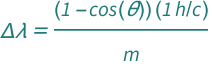symbol description physical quantity
Δ​λ wavelength shift "Wavelength"
θ scattering angle "Angle"
m mass of particle "Mass"

## Examples

Get the resource:

 In:=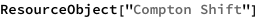Out=Get the formula:

 In:=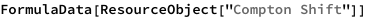Out=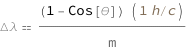Use some values:

 In:=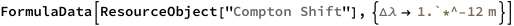Out=